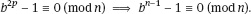Therefore n − 1 is a sum of an even number of terms of the same parity so n − 1 must be even. It follows that 2p divides n − 1. Hence b2p − 1 is a divisor of bn-1 − 1. HoweverTherefore n is a pseudoprime to the base b proving the theorem. □

Although there are infinitely many pseudoprimes they are not that common. It has been shown for example that there are only 21 853 pseudoprimes to the base 2 among the first 25 000 000 000 integers. Hence there is a good chance that if a number, especially a large number, passes a test as a pseudoprime, then it is really a prime. The question becomes how to make this chance, or probability, precise. Lists ...

Get A Course in Mathematical Cryptography now with the O’Reilly learning platform.

O’Reilly members experience books, live events, courses curated by job role, and more from O’Reilly and nearly 200 top publishers.